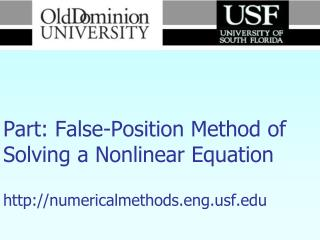DownloadDownload PresentationNumerical Methods Part: False-Position Method of Solving a Nonlinear Equation http://numericalmethods.eng.usf.edu

# Numerical Methods Part: False-Position Method of Solving a Nonlinear Equation http://numericalmethods.eng.usf.edu

Télécharger la présentation## Numerical Methods Part: False-Position Method of Solving a Nonlinear Equation http://numericalmethods.eng.usf.edu

- - - - - - - - - - - - - - - - - - - - - - - - - - - E N D - - - - - - - - - - - - - - - - - - - - - - - - - - -
##### Presentation Transcript

1. Numerical MethodsPart: False-Position Method of Solving a Nonlinear Equationhttp://numericalmethods.eng.usf.edu

2. For more details on this topic • Go to http://numericalmethods.eng.usf.edu • Click on Keyword • Click on False-Position Method of Solving a Nonlinear Equation

3. You are free • to Share – to copy, distribute, display and perform the work • to Remix – to make derivative works

4. Under the following conditions • Attribution — You must attribute the work in the manner specified by the author or licensor (but not in any way that suggests that they endorse you or your use of the work). • Noncommercial — You may not use this work for commercial purposes. • Share Alike — If you alter, transform, or build upon this work, you may distribute the resulting work only under the same or similar license to this one.

5. Chapter 03.06: False-Position Method of Solving a Nonlinear Equation Lecture # 1 Major: All Engineering Majors Authors: Duc Nguyen http://numericalmethods.eng.usf.edu Numerical Methods for STEM undergraduates 4/2/2014 http://numericalmethods.eng.usf.edu 5

6. Introduction (1) In the Bisection method (2) (3) 1 Figure 1 False-Position Method 6 http://numericalmethods.eng.usf.edu

7. False-Position Method Based on two similar triangles, shown in Figure 1, one gets: (4) The signs for both sides of Eq. (4) is consistent, since: http://numericalmethods.eng.usf.edu

8. From Eq. (4), one obtains The above equation can be solved to obtain the next predicted root , as (5) http://numericalmethods.eng.usf.edu

9. The above equation, (6) or (7) http://numericalmethods.eng.usf.edu

10. Step-By-Step False-Position Algorithms 1. Choose and as two guesses for the root such that 2. Estimate the root, 3. Now check the following , then the root lies between (a) If ; then and and , then the root lies between (b) If ; then and and http://numericalmethods.eng.usf.edu

11. , then the root is (c) If Stop the algorithm if this is true. 4. Find the new estimate of the root Find the absolute relative approximate error as http://numericalmethods.eng.usf.edu

12. where = estimated root from present iteration = estimated root from previous iteration If , then go to step 3, 5. else stop the algorithm. Notes: The False-Position and Bisection algorithms are quite similar. The only difference is the formula used to calculate the new estimate of the root shown in steps #2 and 4! http://numericalmethods.eng.usf.edu

13. Example 1 The floating ball has a specific gravity of 0.6 and has a radius of 5.5cm. You are asked to find the depth to which the ball is submerged when floating in water. The equation that gives the depth to which the ball is submerged under water is given by Use the false-position method of finding roots of equations to find the depth to which the ball is submerged under water. Conduct three iterations to estimate the root of the above equation. Find the absolute relative approximate error at the end of each iteration, and the number of significant digits at least correct at the converged iteration. http://numericalmethods.eng.usf.edu

14. Solution From the physics of the problem Figure 2 : Floating ball problem http://numericalmethods.eng.usf.edu

15. Let us assume Hence, http://numericalmethods.eng.usf.edu

16. Iteration 1 http://numericalmethods.eng.usf.edu

17. Iteration 2 Hence, http://numericalmethods.eng.usf.edu

18. Iteration 3 http://numericalmethods.eng.usf.edu

19. Hence, http://numericalmethods.eng.usf.edu

20. Table 1: Root of for False-Position Method. http://numericalmethods.eng.usf.edu

21. The number of significant digits at least correct in the estimated root of 0.062377619 at the end of 4th iteration is 3. http://numericalmethods.eng.usf.edu

22. References • S.C. Chapra, R.P. Canale, Numerical Methods for • Engineers, Fourth Edition, Mc-Graw Hill. http://numericalmethods.eng.usf.edu

23. The End http://numericalmethods.eng.usf.edu

24. Acknowledgement This instructional power point brought to you by Numerical Methods for STEM undergraduate http://numericalmethods.eng.usf.edu Committed to bringing numerical methods to the undergraduate

25. For instructional videos on other topics, go to http://numericalmethods.eng.usf.edu/videos/ This material is based upon work supported by the National Science Foundation under Grant # 0717624. Any opinions, findings, and conclusions or recommendations expressed in this material are those of the author(s) and do not necessarily reflect the views of the National Science Foundation.

26. The End - Really